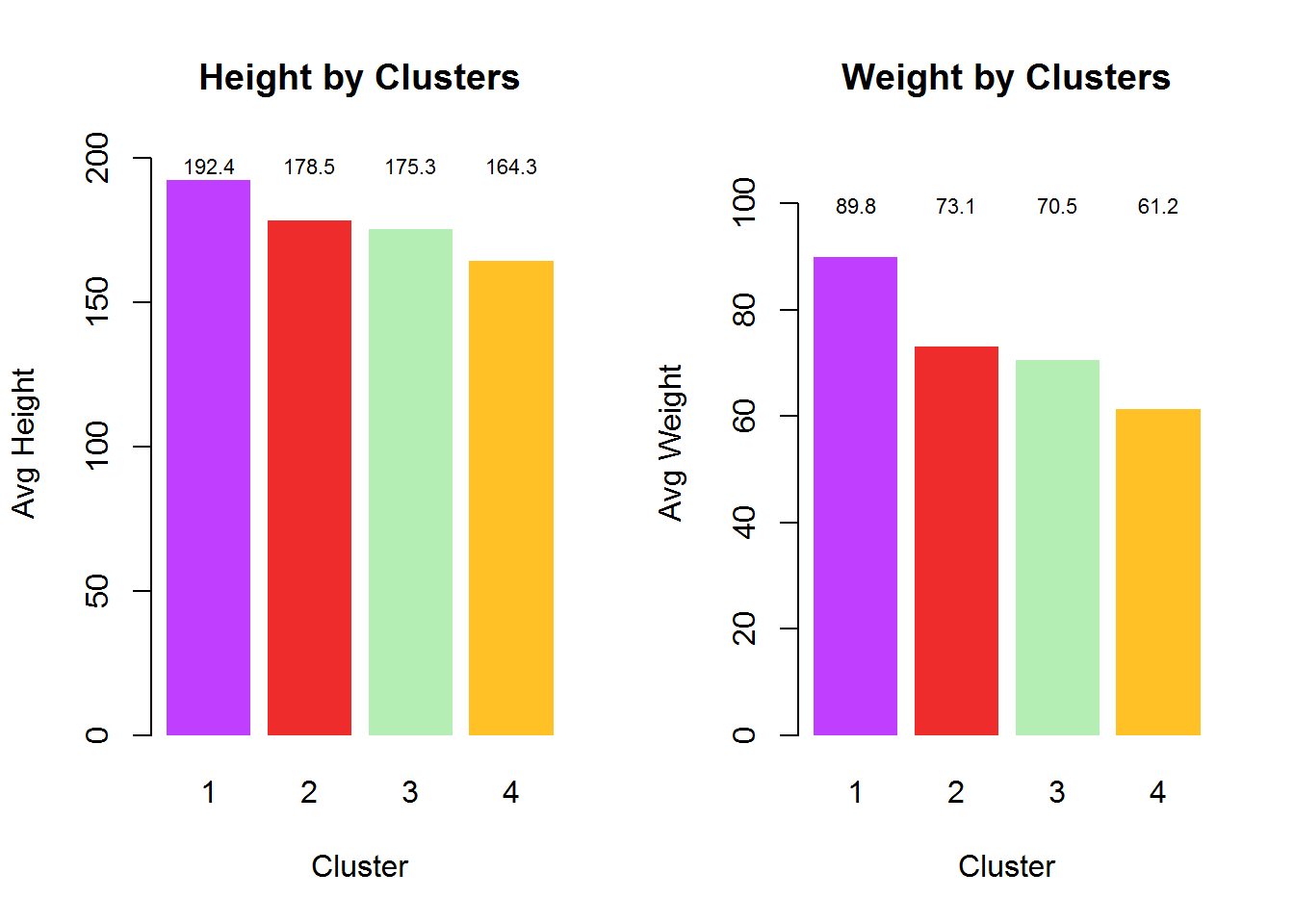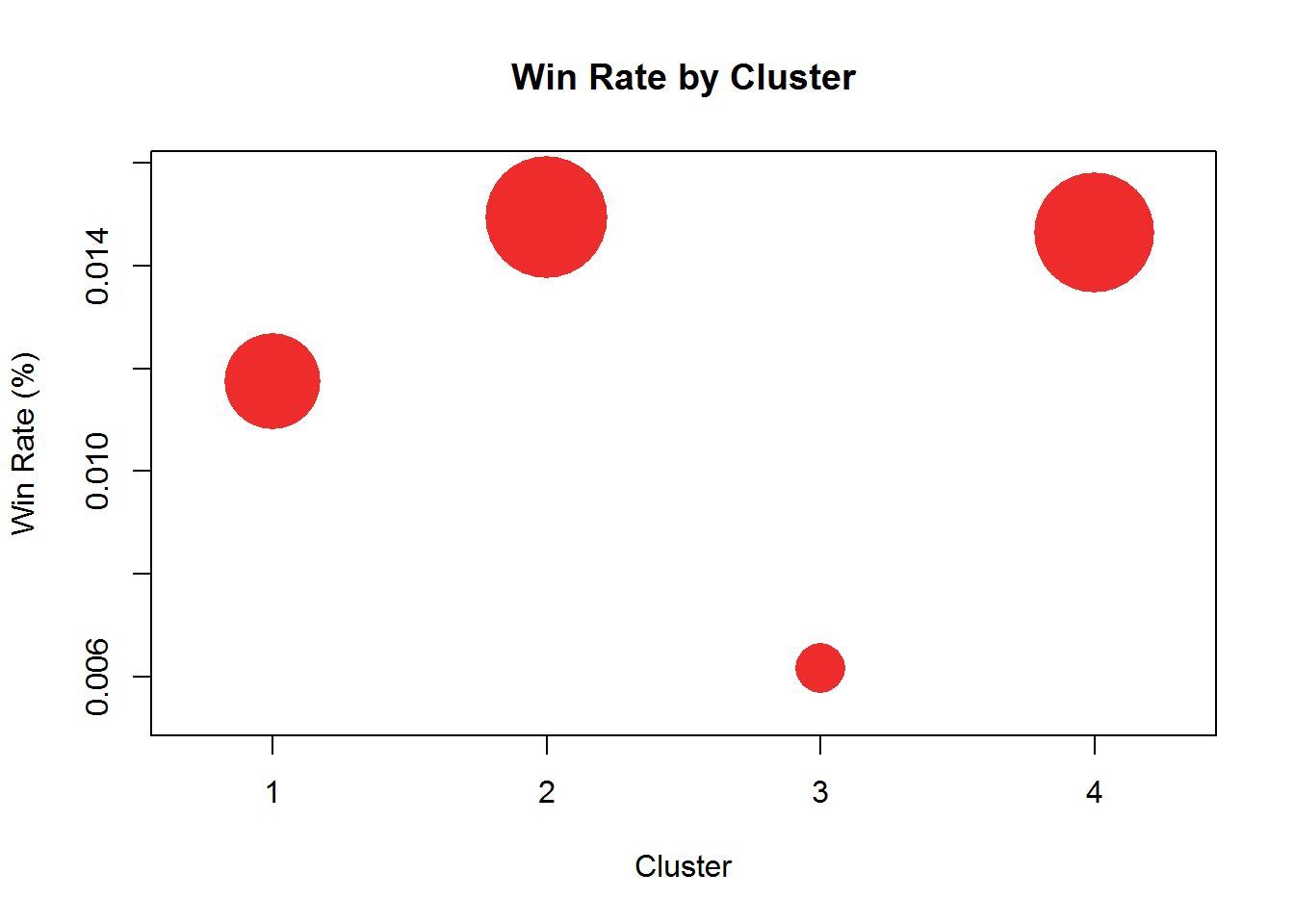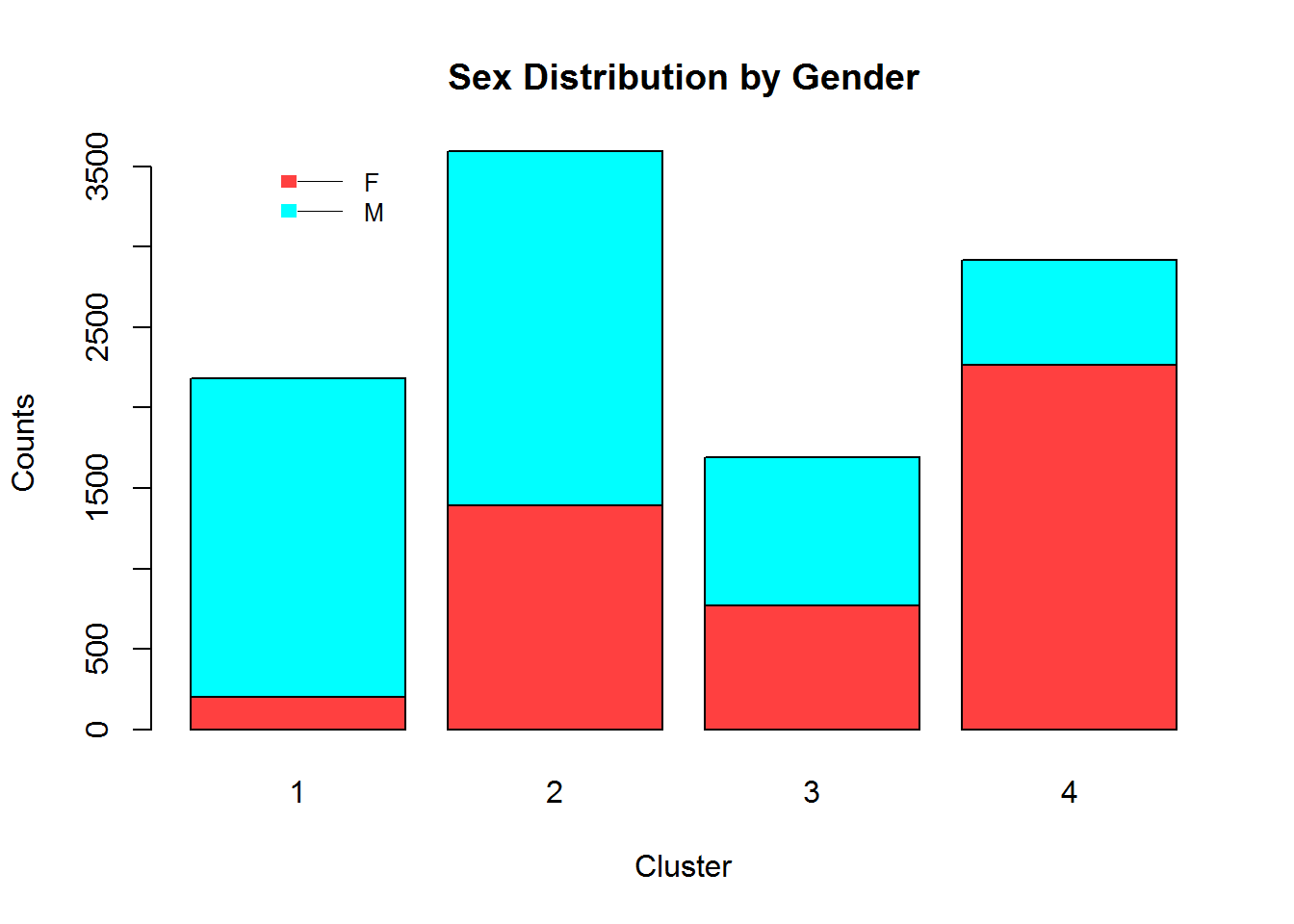# K Means Clustering- Summary Statistics and Visualization

In this post, kmeans function is used for K Means Clustering. Overall approach which is used in K Means Clustering is discussed in the earlier blog.

Using London Athlete Data

setwd("~/training")

londonkm <- kmeans(london[4:5],
centers= 4)

k-means output statistics

kmeans function gives below statistics as standard output.

• Cluster: A vector of integers (from 1:k) indicating the cluster to which each point is allocated.
• Centers: A matrix of cluster centres.
• Totss: The total sum of squares.
• Withinss: Vector of within-cluster sum of squares, one component per cluster.
• tot.withinss: Total within-cluster sum of squares, i.e., sum(withinss).
• Betweenss: The between-cluster sum of squares, i.e.Â totss-tot.withinss.
• Size: The number of points in each cluster.
• Iter: The number of (outer) iterations.
• Ifault: Integer: indicator of a possible algorithm problem Â– for experts.

#### Clustering Performance

We need to have a mechanism to understand and conclude that the clusters created.

SAS Fastclus procedure provides

*   Variable Level R Square
*   Overall R Square
*   Pseudo  F
*   CCC- Cubic Clustering Criterion (CCC).

But kmeans does not provide any of the above statistics. We can write custom functions to calculate the above.

We are showing steps to calculate overall R Square and Pseudo F

Overall R Square is ratio between variance to within Variance. Pseudo F calculation.

#Ratio of between Variance to within cluster analysis
Overall_R_sq <- londonkm$betweenss/londonkm$totss
# Pseudo F
F_Pseudo <- ((londonkm$totss-londonkm$betweenss)/(length(londonkm$withinss)-1))/((londonkm$betweenss)/(sum(londonkm$size)-length(londonkm$withinss)))

Overall_R_sq
##  0.728
F_Pseudo
##  1293

Higher values of overall R Square and Pseudo F Statistics, better (in terms of differential among clusters) is the clusters created.

### Profiling and Visualization

For clustering height and weight of the athletes are used, first we need to compared the heights and weights across clusters to know what type of athletes each cluster has.

par(mfrow=c(1,2))

## Plots on clustering variables
height.m <- aggregate(london$Height, by=list(londonkm$cluster), mean)
names(height.m) <- c("Cluster","Height")

## Bar Plot: Height
cp <-barplot(height=height.m$Height , names.arg=height.m$Cluster,
xlab="Cluster",
ylab="Avg Height",
main="Height by Clusters",
col=c("darkorchid1","firebrick2","darkseagreen2","goldenrod1"),
ylim= c(0,max(height.m$Height)+10 ) , border=NA ) text(cp, (max(height.m$Height)+5), round(height.m$Height, 1),cex=0.7,col="black") weight.m <- aggregate(london$Weight, by=list(londonkm$cluster), mean) names(weight.m ) <- c("Cluster","Weight") ## Bar Plot: Weight cp <-barplot(height=weight.m$Weight ,
names.arg=weight.m$Cluster, xlab="Cluster", ylab="Avg Weight", main="Weight by Clusters", col=c("darkorchid1","firebrick2","darkseagreen2","goldenrod1"), ylim= c(0,max(weight.m$Weight)+20 ) ,
border=NA
)
text(cp, (max(weight.m$Weight)+10), round(weight.m$Weight, 1),cex=0.7,col="black")## Could have used below steps to directly get cluster centers
londonkm$centers ## Age Height ## 1 27.07 192.4 ## 2 23.53 178.5 ## 3 33.52 175.3 ## 4 24.13 164.3 Now, we can leverage other variables for profiling the athletes in each cluster. * Win Rate * Gender Distribution par(mfrow=c(1,1)) # Win Variable Win <- london$Gold>0 | london$Silver | london$Bronze
Win.Cluster <-table(londonkm$cluster,Win) Win.Rate.Cluster <- Win.Cluster[,2]/sum(Win.Cluster[,1],Win.Cluster[,2]) symbols(x=c(1,2,3,4), y=Win.Rate.Cluster, circles=Win.Rate.Cluster, inches=1/3, bg="firebrick2", fg=NULL, xaxt='n', xlab="Cluster", ylab="Win Rate (%)", main="Win Rate by Cluster" ) axis(1, at=c(1,2,3,4), las=1, # Angle of the label labels=c(1,2,3,4), # label of x axis cex.axis=1 #axis label size )## Gender gender <- table(london$Sex,londonkm\$cluster)
par(xpd=TRUE)
barplot(as.matrix(gender),
xlab="Cluster",
ylab="Counts",
col=c("brown1","cyan1"),
main="Sex Distribution by Gender"
)
legend( "topleft",  ## position of the legend
inset=c(0.1,.001), ## allow tweaking of the legend position
legend=row.names(gender), ## legends
border="white", ## legend box line
fill=c("brown1","cyan1"), ## legend colors
lwd=0, ## line width around legend box
box.col="white"  , ## legend box
cex =0.8,  ## size of the legend text and box
bg=NULL  ## legend box color, NULL indicate transparent
)Looking at Plots for Gender/Sex and Win Rat, it is clear that clusters are very distinct on these variables even though these were not considered in cluster creation.